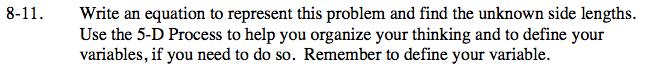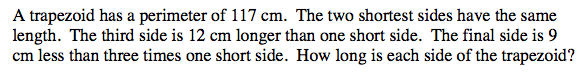Home > CC2 > Chapter 8 > Lesson 8.1.1 > Problem8-11

8-11.
1. Write an equation to represent this problem and find the unknown side lengths.
Use the 5-D Process to help you organize your thinking and to define your variables, if you need to do so. Remember to define your variable.

2. A trapezoid has a perimeter of 117 cm. The two shortest sides have the same length. The third side is 12 cm longer than one short side. The final side is 9 cm less than three times one short side. How long is each side of the trapezoid? Homework Help ✎Perimeter is the sum of the lengths of all the sides.

Create an expression comparing each side with the shortest side, represented by x.

Two Shortest Sides: x
Third Side: 12 + x
Fourth Side: 3x − 9
Perimeter: 117 cm

Shortest Sides: 19 cm
What are the other side lengths?
Be sure to know how to get this length and the other lengths.